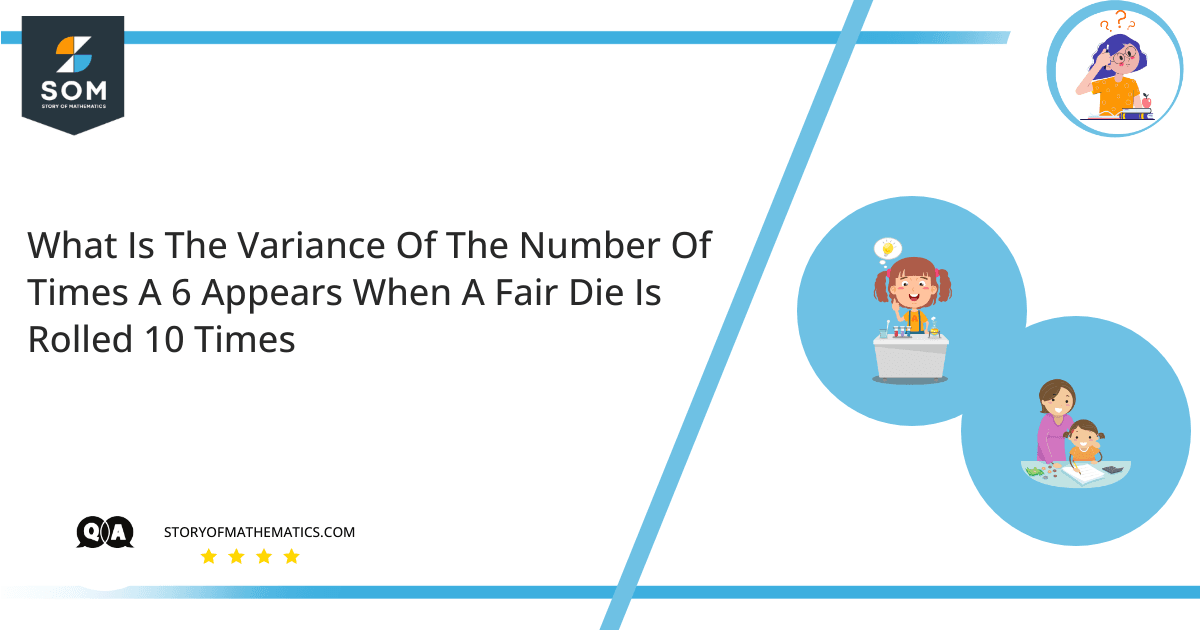# What is the variance of the number of times a 6 appears when a fair die is rolled 10 times?This question aims to find the variance of the number of times a $6$ appears when a fair die is rolled $10$ times.

We are surrounded by randomness. Probability theory is the mathematical concept that enables us to rationally analyze the chance of occurrence of an event. A probability of an event is a number that indicates the likelihood of an event. This number will always be between $0$ and $1$, with $0$ denoting impossibility and $1$ denoting the occurrence of an event.

Variance is a measure of variation. It is calculated by averaging the squared deviations from the mean. The degree of spread in the data set is indicated by variance. The variance will be relatively greater than the mean if the spread of data is large. It is measured in much larger units.

In a binomial distribution, the variance is given by:

$\sigma^2=np(1-p)=npq$

Here, $n$ is the total number of trials and $p$ denotes the probability of success. With this in mind, $q$ is the probability of failure and is equal to $1-p$.

Now, when a fair dice is rolled the number of outcomes is $6$.

And so, the probability of getting a $6$ is $\dfrac{1}{6}$.

Finally, we have the variance as:

$\sigma^2=np(1-p)=(10)\left(\dfrac{1}{6}\right)\left(1-\dfrac{1}{6}\right)$

$=(10)\left(\dfrac{1}{6}\right)\left(\dfrac{5}{6}\right)=\dfrac{25}{18}$

## Example 1

Find the probability of getting a sum of $7$ if two fair dice are rolled.

### Solution

If two dice are rolled, then the number of samples in sample space is $6^2=36$.

Let $A$ be the event of getting a sum of $7$ on both the dice, then:

$A=\{(1,6),(2,5),(3,4),(4,3),(5,2),(6,1)\}$

And $P(A)=\dfrac{6}{36}=\dfrac{1}{6}$

## Example 2

Find the standard deviation of the number of times a $4$ appears when a fair die is rolled $5$ times.

### Solution

Number of samples in sample space $=n(S)=6$

When a fair die is rolled, then the probability of getting a $4$ on a single die is $\dfrac{1}{6}$.

Since the standard deviation is the square root of variance, therefore:

$\sigma=\sqrt{\sigma^2}=\sqrt{npq}$

Here, $n=5$, $p=\dfrac{1}{6}$ and $q=1-p=\dfrac{5}{6}$.

So, $\sigma=\sqrt{(5)\left(\dfrac{1}{6}\right)\left(\dfrac{5}{6}\right)}$

$=\sqrt{\dfrac{25}{36}}$

$=\dfrac{5}{6}$

$=0.833$